# Scatterplots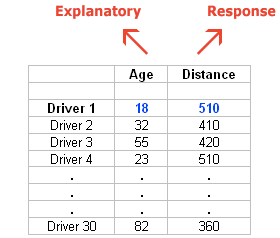# Interpreting Scatterplots

• Direction: Positive or Negative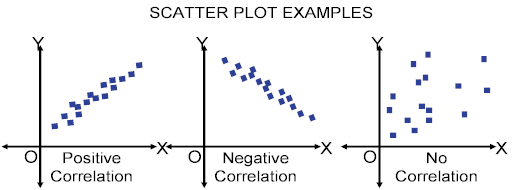• Form: Linear or Non-linear• Strength: Weak, Moderate or Strong• Example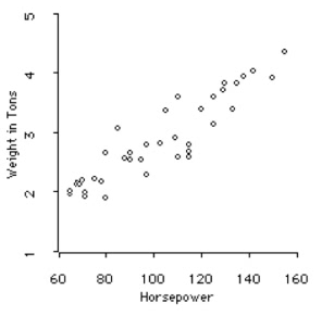• Positive, linear, strong relationship between horsepower and weight in tons.

# Correlation Coefficient (r)# Least Squares Regression Line• A regression line is a line that describes how y changes as x changes

• Can be used to predict the value of y for a given value of x

• Called the Least Squares regression line because it make the smallest sum of squares

• LSRL will always run through the point (mean of x, mean of y)

• Formulas (hat = predicted)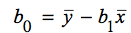• Remember to note what x and y are

• Calculation

• Input data• STAT➡️CALC➡️ 4:LinReg(ax+b)

• LinReg(ax+b) L1, L2• Catalog (2ND + 0) ➡️ DiagnosticOn• Do LinReg again to display r

![ТИ-84 PIus S'tver Editj(n ф TEns [NSTRUMENTS гоамдтп сам: а ](./media/image58.png)

# Coefficient of Determination

• R^2=r^2

• Coefficient of Determination = (Correlation Coefficient)^2

• Percent of the change in y that is explained by the change by the change in x / least squares regression line

• From the previous example, 41.9% of the change in y can be explained by the change in x

# Residuals (≈error)

• Residuals = observed/actual y - predicted y

• Resid = y - y hat

• Resid < 0: Overpredicted

• Resid > 0: Underpredicted• Residual Plot• no pattern = good fit
• Example
X 3 1 1.5 6 2
Y 10 3 14 15 6
Y hat 10.1 6.76 7.60 15.11 8.43
Residuals -0.1 -3.76 6.4 -0.11 -2.43
• Calculator

• Type the regression equation in L3 (y hat)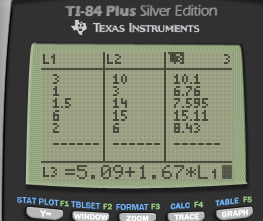• L4 = L2 - L3• Graph L1, L4 (Residuals)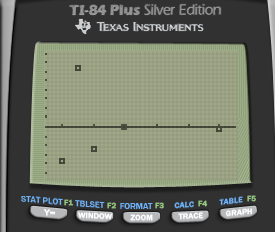# Examples

• At the summer school, one of Sarah's teachers told her that you can determine air temperature from the number of cricket chirps
1. What is the explanatory variable, and what it the response variable

• Explanatory/independent variable: cricket chirps

• Response/dependent variable: air temperature

2. To determine a formula, Sarah collected data on temperature and number of chirps per minute on 14 occasions. She entered the data into her calculator and did 2-Var Stats. Here are some results. Use this information to find the equation of the least-squares regression line

Xbar 165.8
Sx 32.0
Ybar 76.83
Sy 9.23
r 0.361
• b= r * Sy / Sx = 0.104

• Ybar = a + b*Xbar

• a = Ybar - b*Xbar = 59.57

• Yhat = 59.57 + 0.14 * x

• Where y = air temperature, and x = cricket chirps

1. One of Sarah's data points was recorded on a particularly hot day (95F). She counted 2432 cricket chirps in one minute. What is the residual for this data point?

• Residual = Y - Yhat = 95 - (59.57 + 0.104 * 2432) = -217.498